# Bisectors in a Triangle

### Perpendicular bisector

The perpendicular bisector of a side of a triangle is a line perpendicular to the side and passing through its midpoint.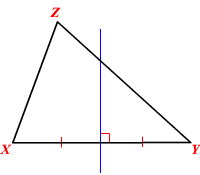The three perpendicular bisectors of the sides of a triangle meet in a single point, called the circumcenter . A point where three or more lines intersect is called a point of concurrency. So, the circumcenter is the point of concurrency of perpendicular bisectors of a triangle.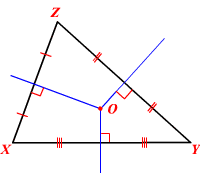Here, $O$ is the circumcenter of $\Delta XYZ$ .

The circumcenter is equidistant from the vertices of the triangle.  (See circumcenter theorem.) That is, $XO=YO=ZO$ . The circle drawn with the circumcenter as the center and the radius equal to this distance passes through all the three vertices and is called circumcircle . This is the smallest circle that the triangle can be inscribed in.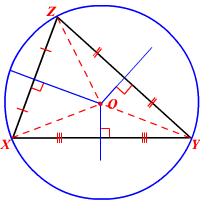The circumcenter lies inside the triangle for acute triangles, on the hypotenuse for right triangles and lies outside the triangle for obtuse triangles. The circumcenter coincides with the midpoint of the hypotenuse if it is an isosceles right triangle.

Example 1:

Natha, Hiren and Joe’s homes represent three non-collinear points on a coordinate plane. If they want to meet at a common place such that each one will have to travel the same distance from their homes, how will you decide the meeting point?

Since the points representing the homes are non-collinear, the three points form a triangle.

Now, if you consider the circumcenter of the triangle, it will be equidistant from the vertices. That is, if the circumcenter of the triangle formed by the three homes is chosen as the meeting point, then each one will have to travel the same distance from their home.

Example 2:

Find the value of $x$ .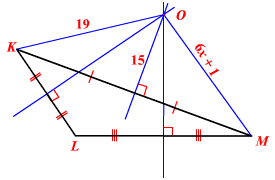Here, $O$ is the point of concurrency of the three perpendicular bisectors of the sides of $\Delta KLM$ .

So, $O$ is the circumcenter of the triangle.

The circumcenter is equidistant from the vertices. Then, $OM=OK$ .

That is, $6x+1=19$ .

Solve for $x$ .

$\begin{array}{l}6x+1-1=19-1\\ 6x=18\\ \frac{6x}{6}=\frac{18}{6}\\ x=3\end{array}$

### Angle bisector

The angle bisector of an angle of a triangle is a straight line that divides the angle into two congruent angles.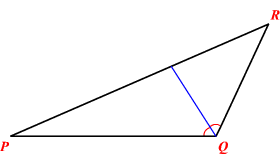The three angle bisectors of the angles of a triangle meet in a single point, called the incenter .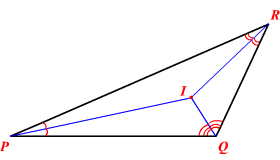Here, $I$ is the incenter of $\Delta PQR$ .

The incenter is equidistant from the sides of the triangle.  That is, $PI=QI=RI$ . The circle drawn with the incenter as the center and the radius equal to this distance touches all three sides and is called incircle or the inscribed circle of the triangle. This circle is the largest circle that will fit inside the triangle.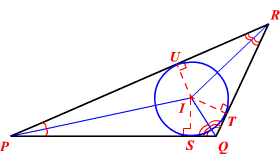For an equilateral triangle the incenter and the circumcenter will be the same.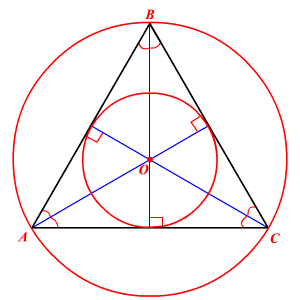Example 3:

Misty has a triangular piece of backyard where she wants to build a swimming pool. How can she find the largest circular pool that can be built there?

The largest possible circular pool would have the same size as the largest circle that can be inscribed in the  triangular backyard. The largest circle that can be inscribed in a triangle is incircle. This can be determined by finding the point of concurrency of the angle bisectors of each corner of the backyard and then making a circle with this point as center and the shortest distance from this point to the boundary as radius.

Example 4:

Find the length $JO$ .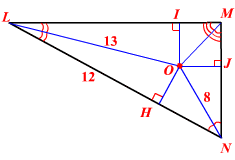Here, $O$ is the point of concurrency of the three angle bisectors of $\Delta LMN$ and therefore is the incenter. The incenter is equidistant from the sides of the triangle. That is, $JO=HO=IO$ .

We have the measures of two sides of the right triangle $\Delta HOL$ , so it is possible to find the length of the third side.

Here, $O$ is the point of concurrency of the three angle bisectors of $\Delta LMN$ and therefore is the incenter. The incenter is equidistant from the sides of the triangle. That is, $JO=HO=IO$ .

We have the measures of two sides of the right triangle $\Delta HOL$ , so it is possible to find the length of the third side.

Use the Pythagorean Theorem to find the length $HO$ .

$\begin{array}{l}HO=\sqrt{{\left(LO\right)}^{2}-{\left(HL\right)}^{2}}\\ \text{\hspace{0.17em}}\text{\hspace{0.17em}}\text{\hspace{0.17em}}\text{\hspace{0.17em}}\text{\hspace{0.17em}}\text{\hspace{0.17em}}\text{\hspace{0.17em}}\text{\hspace{0.17em}}\text{\hspace{0.17em}}=\sqrt{{13}^{2}-{12}^{2}}\\ \text{\hspace{0.17em}}\text{\hspace{0.17em}}\text{\hspace{0.17em}}\text{\hspace{0.17em}}\text{\hspace{0.17em}}\text{\hspace{0.17em}}\text{\hspace{0.17em}}\text{\hspace{0.17em}}\text{\hspace{0.17em}}=\sqrt{169-144}\\ \text{\hspace{0.17em}}\text{\hspace{0.17em}}\text{\hspace{0.17em}}\text{\hspace{0.17em}}\text{\hspace{0.17em}}\text{\hspace{0.17em}}\text{\hspace{0.17em}}\text{\hspace{0.17em}}\text{\hspace{0.17em}}=\sqrt{25}\\ \text{\hspace{0.17em}}\text{\hspace{0.17em}}\text{\hspace{0.17em}}\text{\hspace{0.17em}}\text{\hspace{0.17em}}\text{\hspace{0.17em}}\text{\hspace{0.17em}}\text{\hspace{0.17em}}\text{\hspace{0.17em}}=5\end{array}$

Since $JO=HO$ , the length $JO$ also equals $5$ units.